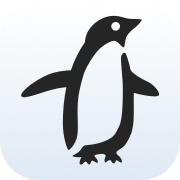FreeWheel Linear Programming Interface for Python

# FlipyFlipy is a Python linear programming interface library, originally developed by FreeWheel. It currently supports Gurobi and CBC as the backend solver.

To use Gurobi, make sure you have a Gurobi license file, and gurobipy is installed in your Python environment. You can find details from Gurobi’s documentation.

Flipy requires Python 3.6 or newer.

## Installation

The latest offical version of Flipy can be installed with pip:

pip install flipy


The latest development version can be get with Git:

git clone https://github.com/freewheel/flipy.git
cd flipy
python setup.py install


## Quickstart

Here is a simple example for Flipy:

import flipy

# 1 <= x <= 3.5
x = flipy.LpVariable('x', low_bound=1, up_bound=3.5)
# 2 <= y <= 4
y = flipy.LpVariable('y', low_bound=2, up_bound=4)

# 5x + y <= 12
lhs = flipy.LpExpression('lhs', {x: 2.5, y: 1})
rhs = flipy.LpExpression('rhs', constant=12)
constraint = flipy.LpConstraint(lhs, 'leq', rhs)

# maximize: 3x + 2y
objective = flipy.LpObjective('test_obj', {x: 3, y: 2}, sense=flipy.Maximize)
problem = flipy.LpProblem('test', objective, [constraint])

solver = flipy.CBCSolver()
status = solver.solve(problem)


## Get the solution

After solving, a status is returned to indicate whether the solver has found a optimal solution for the problem:

print(status)
# <SolutionStatus.Optimal: 1>


The objective value can be retrieved with objective.evaluate():

print(objective.evaluate())
# 17.6


The value of variables can be retrieved with .evaluate() as well:

print(x.evaluate())
# 3.2
print(y.evaluate())
# 4.0


## Project details

This version0.0.50.0.40.0.30.0.20.0.1

Uploaded source
Uploaded py3